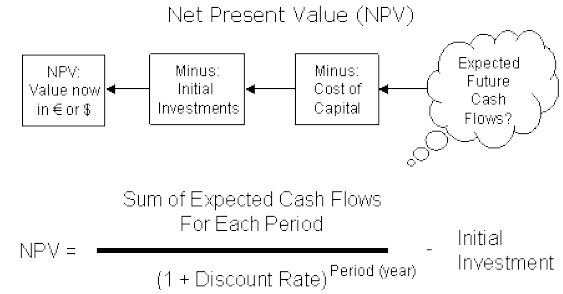# Investment Under Certainty

Capital Budgeting is the process by which the firm decides which long-term investments to make. Capital Budgeting projects, i.e., potential long-term investments, are expected to generate cash flows over several years.

Capital Budgeting also explains the decisions in which all the incomes and expenditures are covered. These decisions involve all inflows and outflows of funds of an undertaking for a particular period of time.

Capital Budgeting techniques under certainty can be divided into the following two groups −

Non Discounted Cash Flow

• Pay Back Period
• Accounting Rate of Return (ARR)

Discounted Cash Flow

• Net Present Value (NPV)
• Profitability Index (PI)
• Internal Rate of Return (IRR)

The payback period (PBP) is the traditional method of capital budgeting. It is the simplest and perhaps the most widely used quantitative method for appraising capital expenditure decision; i.e. it is the number of years required to recover the original cash outlay invested in a project.

## Non-Discounted Cash Flow

Non-discounted cash flow techniques are also known as traditional techniques.

### Pay Back Period

Payback period is one of the traditional methods of budgeting. It is widely used as quantitative method and is the simplest method in capital expenditure decision. Payback period helps in analyzing the number of years required to recover the original cash outlay invested in a particular project. The formula widely used to calculate payback period is −

PBP =
Initial Investment / Constant annual cash inflow

PBP is a cost effective and easy to calculate method. It is simple to use and does not require much of the time for calculation. It is more helpful for short term earnings.

### Accounting Rate of Return (ARR)

The ARR is the ratio after tax profit divided by the average investment. ARR is also known as return on investment method (ROI). Following formula is usually used to calculate ARR −

ARR =
Average annual profit after tax / Average investment
×
100

The average profits after tax are obtained by adding up the profit after tax for each year and dividing the result by the number of years.

ARR is simple to use and as it is based on accounting information, it is easily available. ARR is usually used as a performance evaluation measure and not as a decision making tool as it does not use cash flow information.

## Discounted Cash Flow Techniques

Discounted cash flow techniques consider time value of money and are therefore also known as modern techniques.

### Net Present Value (NPV)

The net present value is one of the discounted cash flow techniques. It is the difference between the present value of future cash inflows and the present value of the initial outlay, discounted at the firm’s cost of capital. It recognizes the cash flow streams at different time intervals and can be computed only when they are expressed in terms of common denominator (present value). Present value is calculated by determining an appropriate discount rate. NPV is calculated with the help of equation.

NPV = Present value of cash inflows − Initial investment.NPV is considered as the most appropriate measure of profitability. It considers all the years of cash flow, and recognizes the time value for money. It is an absolute measure of profitability that means it gives output in terms of absolute amount. The NPVs of the projects can be added together which is not possible in other methods.

## Profitability Index (PI)

Profitability index method is also known as benefit cost ratio as numerator measures benefits and denominator measures cost like the NPV approach. It is the ratio obtained by dividing the present value of future cash inflows by the present value of cash outlays. Mathematically it is defined as −

PI =
Present value of cash inflow / Initial cash outlay

In a capital rationing situation, PI is a better evaluation method as compared to NPV method. It considers the time value of money along cash flows generated by the project.

Present Cash Value
Year Cash Flows @ 5% Discount @ 10% Discount
0 \$ -10,000.00 \$ -10,000.00 \$ -10,000.00
1 \$ 2,000.00 \$ 1,905.00 \$ 1,818.00
2 \$ 2,000.00 \$ 1,814.00 \$ 1,653.00
3 \$ 2,000.00 \$ 1,728.00 \$ 1,503.00
4 \$ 2,000.00 \$ 1,645.00 \$ 1,366.00
5 \$ 5,000.00 \$ 3,918.00 \$ 3,105.00
Total \$ 1,010.00 \$ -555.00
Profitability Index (5%) =
\$11010 / \$10000
= 1.101
Profitability Index (10%) =
\$9445 / \$10000
= .9445

## Internal Rate of Return (IRR)

Internal rate of return is also known as yield on investment. IRR depends entirely on the initial outlay of the projects which are evaluated. It is the compound annual rate of return that the firm earns, if it invests in the project and receives the given cash inflows. Mathematically IRR is determined by the following equation −

IRR = T t=1
Ct / (1 + r)t
− 1c0

Where,

R = The internal rate of return

Ct = Cash inflows at t period

C0 = Initial investment

Example −

Internal Rate of Return
Opening Balance -100,000
Year 1 Cash Flow 110000
Year 2 Cash Flow 113000
Year 3 Cash Flow 117000
Year 4 Cash Flow 120000
Year 5 Cash Flow 122000
Proceeds from Sale 1100000
IRR 9.14%